Courses

# Thyristor Commutation Techniques

## 10 Questions MCQ Test Topicwise Question Bank for Electronics Engineering | Thyristor Commutation Techniques

Description
This mock test of Thyristor Commutation Techniques for Electrical Engineering (EE) helps you for every Electrical Engineering (EE) entrance exam. This contains 10 Multiple Choice Questions for Electrical Engineering (EE) Thyristor Commutation Techniques (mcq) to study with solutions a complete question bank. The solved questions answers in this Thyristor Commutation Techniques quiz give you a good mix of easy questions and tough questions. Electrical Engineering (EE) students definitely take this Thyristor Commutation Techniques exercise for a better result in the exam. You can find other Thyristor Commutation Techniques extra questions, long questions & short questions for Electrical Engineering (EE) on EduRev as well by searching above.
QUESTION: 1

### To turn-off or commutate a thyristor

Solution:

To turn-off a thyristor, anode current must be reduced below holding current and a reverse bias must be applied across thyristor for a finite period of time. If all these two conditions are not met simultaneously then forced commutation can be used to turn-off the thyristor.

QUESTION: 2

Solution:
QUESTION: 3

### Turn-off time of a thyristor

Solution:
QUESTION: 4

Turn-on time (ton) of an SCR is related to its turn-off time (toff) in which of the following way?

Solution:

The turn-on time of an SCR depends on time constant (L/R) of the load.

QUESTION: 5

Assertion (A) : It is necessary to keep the thyristor reverse biased for a finite period before a forward anode voltage can be reapplied.
Reason (R) : Once the SCR starts conducting an appreciable forward current, the gate has no control on it and the device can be brought back to the blocking state only by reducing the forward current level below that of the holding current.

Solution:

It is necessary to keep the thyristor reverse biased for a finite period before a forward anode voltage can be reapplied because if a forward voltage is applied immediately after reducing the anode current to zero, it will not block the forward voltage and will start conducting again, although it is not triggered by a gate pulse. Reason is also a true statement but not the correct explanation of assertion.

QUESTION: 6

Match List-I (Commutation techniques) with List-ll (Alternative name) and select the correct answer using the codes given below the lists:
List-I
A. Class-E
B. Class-F
C. Class-B, C and D
D. Class-A

List-ll
1. Forced commutation
2. Load commutation
3. External-pulse commutation
4. Line commutation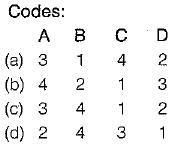Solution:
QUESTION: 7

Consider the following statements associated with various commutation techniques of a thyristor:
1. In forced commutation, the commutating components L and C carry load current continuously.
2. Forced commutation is usually employed in dc choppers.
3. In load commutation, L and Care connected in parallel with the load or C in series with the load.
4. Load commutation is employed in series inverters.
5. In class-C commutation, the main thyristor that is to be commutated is connected in parallel with the load.

Which of the statements given above are correct?

Solution:
• In forced commutation, the commutating components L and C do not carry load current continuously.
• Forced commutation is usually employed in dc choppers because natural current zero is not available in the circuits containing dc choppers.
• In load commutation. L and Care connected in series with the load or C in parallel with the load such that overall load circuit is underdamped.
• Load commutation is employed in series inverters.
•  In class-C commutation, the main thyristor that is to be commutated is connected in series with the load while the complementary thyristor is connected in parallel with the main thyristor as shown below.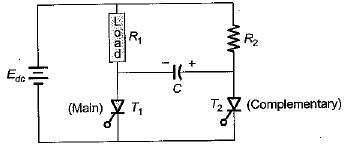Thus, statements 2 and 4 are only correct.

QUESTION: 8

For the class-B commutation circuit shown below, the initial voltage across capacitor is 230 volt. For a constant load current of 300 A, the conduction time for the auxiliary thyristor is approximately equal to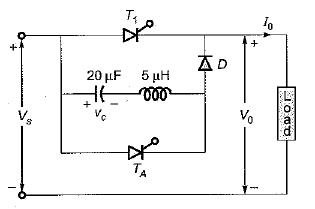Solution:

Given, L = 5μH, C = 20μF
Resonant frequency,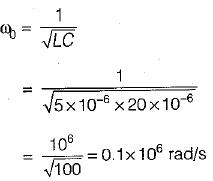∴ Conduction time for auxiliary thyristor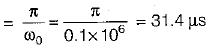QUESTION: 9

For the circuit shown below, the peak value of the current through thyristors T1 and T2 are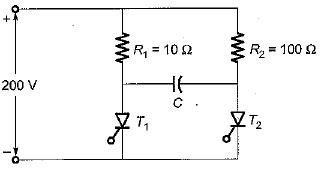Solution:

Given, Vs = 200 V, R= 10 Ω, and R2 = 100 Ω
Given circuit represents a class-C commutation circuit.
The peak value of current through thyristor T1 is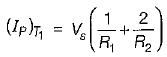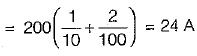The peak value of current through thyristor T2 is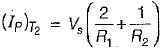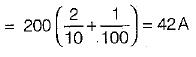QUESTION: 10

The SCR in the circuit shown below is forced commutated by a circuitry not shown in the figure. The SCR has minimum charging current of 5 mA to turn it on and its junction capacitance is 25 pF.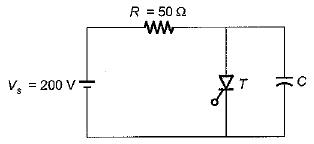Solution:

Under steady-state condition, voltage across capacitor is Vs = 200 V and SCR conducts having a current through it equal to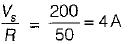When the SCR is forced commutated, the capacitor voltage at time t is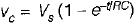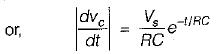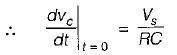...(i)
Let Cj be the junction capacitance of SCR. Now, when SCR get turned-on, the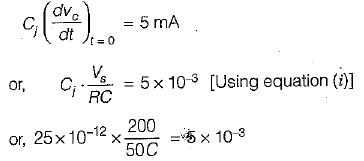or,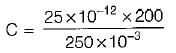= 0.02 μF
Thus, the minimum value of C so that SCR does not get turn-on is 0.02 μF.
If C > 0.02 μF, SCR will be turn-on.